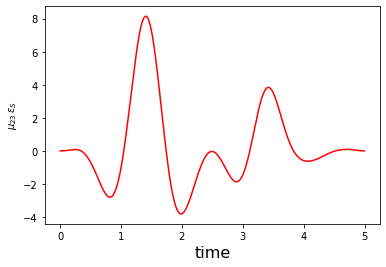# Optimization of a Dissipative State-to-State Transfer in a Lambda System¶

In :
# NBVAL_IGNORE_OUTPUT
import os
import qutip
import numpy as np
import scipy
import matplotlib
import matplotlib.pylab as plt
import krotov
import qutip
from qutip import Qobj
import pickle
%watermark -v --iversions

numpy            1.16.4
scipy            1.2.1
krotov           0.3.0+dev
qutip            4.3.1
matplotlib       3.1.0
matplotlib.pylab 1.16.4
CPython 3.7.3
IPython 7.5.0


$\newcommand{tr}{\operatorname{tr}} \newcommand{diag}{\operatorname{diag}} \newcommand{abs}{\operatorname{abs}} \newcommand{pop}{\operatorname{pop}} \newcommand{aux}{\text{aux}} \newcommand{opt}{\text{opt}} \newcommand{tgt}{\text{tgt}} \newcommand{init}{\text{init}} \newcommand{lab}{\text{lab}} \newcommand{rwa}{\text{rwa}} \newcommand{bra}{\langle#1\vert} \newcommand{ket}{\vert#1\rangle} \newcommand{Bra}{\left\langle#1\right\vert} \newcommand{Ket}{\left\vert#1\right\rangle} \newcommand{Braket}{\left\langle #1\vphantom{#2} \mid # 2\vphantom{#1}\right\rangle}¶ \newcommand{Ketbra}{\left\vert#1\vphantom{#2} \right\rangle \hspace{-0.2em} \left\langle #2\vphantom{#1} \right\vert} \newcommand{op}{\hat{#1}} \newcommand{Op}{\hat{#1}} \newcommand{dd}{\,\text{d}} \newcommand{Liouville}{\mathcal{L}} \newcommand{DynMap}{\mathcal{E}} \newcommand{identity}{\mathbf{1}} \newcommand{Norm}{\lVert#1\rVert} \newcommand{Abs}{\left\vert#1\right\vert} \newcommand{avg}{\langle#1\rangle} \newcommand{Avg}{\left\langle#1\right\rangle} \newcommand{AbsSq}{\left\vert#1\right\vert^2} \newcommand{Re}{\operatorname{Re}} \newcommand{Im}{\operatorname{Im}} \newcommand{toP}{\omega{12}} \newcommand{toS}{\omega{23}}$

This example illustrates the use of Krotov's method with a non-Hermitian Hamiltonian. It considers the same system as the previous example, a transition $\ket{1} \rightarrow \ket{3}$ in a three-level system in a $\Lambda$-configuration. However, here we add a non-Hermitian decay term to model loss from the intermediary level $\ket{2}$.

## The effective Hamiltonian¶

We consider the system as in the following diagram:with the Hamiltonian

$$\Op{H}_{\text{lab}} = \begin{pmatrix} E_1 & -\mu_{12} \epsilon_P(t) & 0 \\ -\mu_{12} \epsilon_P(t) & E_2 & - \mu_{23} \epsilon_S(t) \\ 0 & -\mu_{23} \epsilon_S(t) & E_2 \end{pmatrix}$$

in the lab frame.

However, we now also include that the level $\ket{2}$ decays incoherently. This is the primary motivation of the STIRAP scheme: through destructive interference it can keep the dynamics in a "dark state" where the population is transferred from $\ket{1}$ to $\ket{3}$ without ever populating the $\ket{2}$ state. A rigorous treatment would be to include the dissipation as a Lindblad operator, and to simulate the dynamics and perform the optimization in Liouville space. The Lindblad operator for spontaneous decay from level $\ket{2}$ with decay rate $2\gamma$ is $\Op{L} = \sqrt{2\gamma} \Ketbra{1}{2}$. However, this is numerically expensive. For the optimization, it is sufficient to find a way to penalize population in the $\ket{2}$ state.

Motivated by the Monte-Carlo Wave Function (MCWF) method, we define the non-Hermitian effective Hamiltonian

$$\Op{H}_{\text{eff}} = \Op{H}_{\text{lab}} - \frac{i}{2} \Op{L}^{\dagger} \Op{L}$$

In explicit form, this is

$$\Op{H}_{\text{eff}} = \begin{pmatrix} E_1 & -\mu_{12} \epsilon_P(t) & 0 \\ -\mu_{12} \epsilon_P(t) & E_2 - i \gamma & - \mu_{23} \epsilon_S(t) \\ 0 & -\mu_{23} \epsilon_S(t) & E_2 \end{pmatrix}$$

The only change is that the energy of level $\ket{2}$ now has an imaginary part $-\gamma$, which causes an exponential decay of any population amplitude in $\ket{2}$, and thus a decay in the norm of the state. In the MCWF, this decay of the norm is used to track the probability that quantum jump occurs (otherwise, the state is re-normalized). Here, we do not perform quantum jumps or renormalize the state. Instead, we use the decay in the norm to steer the optimization. Using the functional

$$J_{T, \text{re}} = 1 - \Re{\Braket{\Psi(T)}{\Psi^{\tgt}}}$$

to be minimized, we find that the value of the functional increases if $\Norm{\ket{\Psi(T)}} < 1$. Thus, population in $\ket{2}$ is penalized, without any significant numerical overhead.

The decay rate $2\gamma$ does not necessarily need to correspond to the actual physical lifetime of the $\ket{2}$ state: we can choose an artificially high decay rate to put a stronger penalty on the $\ket{2}$ level. Or, if the physical decay is so strong that the norm of the state reaches effectively zero, we could decrease $\gamma$ to avoid numerical instability. The use of a non-Hermitian Hamiltonian with artificial decay is generally a useful trick to penalize population in a subspace.

The new non-Hermitian decay term remains unchanged when we make the rotating wave approximation. The RWA Hamiltonian now reads

$$\Op{H}_{\text{RWA}} = \begin{pmatrix} \Delta_P & -\frac{1}{2} \Omega_P(t) & 0 \\ -\frac{1}{2} \Omega_P^*(t) & -i \gamma & -\frac{1}{2} \Omega_S(t) \\ 0 & -\frac{1}{2} \Omega_S^*(t) & \Delta_S \end{pmatrix}\,,$$

with complex control fields $\Omega_P(t)$ and $\Omega_S(t)$, see the previous example. Again, we split these complex pulses into an independent real and imaginary part for the purpose of optimization.

The guess controls are

In :
def Omega_P1(t, args):
"""Guess for the real part of the pump pulse"""
Ω0 = 5.0
return Ω0 * krotov.shapes.blackman(t, t_start=2.0, t_stop=5.0)

def Omega_P2(t, args):
"""Guess for the imaginary part of the pump pulse"""
return 0.0

def Omega_S1(t, args):
"""Guess for the real part of the Stokes pulse"""
Ω0 = 5.0
return Ω0 * krotov.shapes.blackman(t, t_start=0.0, t_stop=3.0)

def Omega_S2(t, args):
"""Guess for the imaginary part of the Stokes pulse"""
return 0.0


and the Hamiltonian is instantiated as

In :
def hamiltonian(E1=0.0, E2=10.0, E3=5.0, omega_P=9.5, omega_S=4.5, gamma=0.5):
"""Lambda-system Hamiltonian in the RWA"""

# detunings
ΔP = E1 + omega_P - E2
ΔS = E3 + omega_S - E2

H0 = Qobj([[ΔP, 0.0, 0.0], [0.0, -1j * gamma, 0.0], [0.0, 0.0, ΔS]])

HP_re = -0.5 * Qobj([[0.0, 1.0, 0.0], [1.0, 0.0, 0.0], [0.0, 0.0, 0.0]])
HP_im = -0.5 * Qobj([[0.0, 1.0j, 0.0], [-1.0j, 0.0, 0.0], [0.0, 0.0, 0.0]])

HS_re = -0.5 * Qobj([[0.0, 0.0, 0.0], [0.0, 0.0, 1.0], [0.0, 1.0, 0.0]])
HS_im = -0.5 * Qobj([[0.0, 0.0, 0.0], [0.0, 0.0, 1.0j], [0.0, -1.0j, 0.0]])

return [
H0,
[HP_re, Omega_P1],
[HP_im, Omega_P2],
[HS_re, Omega_S1],
[HS_im, Omega_S2],
]

In :
H = hamiltonian()


We check the hermiticity of the Hamiltonian:

In :
print("H0 is Hermitian: " + str(H.isherm))
print("H1 is Hermitian: "+ str(
H.isherm
and H.isherm
and H.isherm
and H.isherm))

H0 is Hermitian: False
H1 is Hermitian: True


## Define the optimization target¶

We optimize for the phase-sensitive transition $\ket{1} \rightarrow \ket{3}$. As we are working in the rotating frame, the target state must be adjusted with an appropriate phase factor:

In :
ket1 = qutip.Qobj(np.array([1.0, 0.0, 0.0]))
ket2 = qutip.Qobj(np.array([0.0, 1.0, 0.0]))
ket3 = qutip.Qobj(np.array([0.0, 0.0, 1.0]))

def rwa_target_state(ket3, E2=10.0, omega_S=4.5, T=5):
return np.exp(1j * (E2 - omega_S) * T) * ket3

psi_target = rwa_target_state(ket3)


The objective is now instantiated as

In :
objectives = [krotov.Objective(initial_state=ket1, target=psi_target, H=H)]
objectives

Out:
[Objective[|Ψ₀(3)⟩ to |Ψ₁(3)⟩ via [A₀[3,3], [H₁[3,3], u₁(t)], [H₂[3,3], u₂(t)], [H₃[3,3], u₃(t)], [H₄[3,3], u₄(t)]]]]

## Simulate dynamics under the guess field¶

We use a time grid with 500 steps between $t=0$ and $T=5$:

In :
tlist = np.linspace(0, 5, 500)


We propagate once for the population dynamics, and once to obtain the propagated states for each point on the time grid:

In :
proj1 = qutip.ket2dm(ket1)
proj2 = qutip.ket2dm(ket2)
proj3 = qutip.ket2dm(ket3)

guess_dynamics = objectives.propagate(
tlist, propagator=krotov.propagators.expm, e_ops=[proj1, proj2, proj3]
)
guess_states = objectives.propagate(
tlist, propagator=krotov.propagators.expm
)

In :
def plot_population(result):
fig, ax = plt.subplots()
ax.axhline(y=1.0, color='black', lw=0.5, ls='dashed')
ax.axhline(y=0.0, color='black', lw=0.5, ls='dashed')
ax.plot(result.times, result.expect, label='1')
ax.plot(result.times, result.expect, label='2')
ax.plot(result.times, result.expect, label='3')
ax.legend()
ax.set_xlabel('time')
ax.set_ylabel('population')
plt.show(fig)

def plot_norm(result):

state_norm = lambda i: result.states[i].norm()
states_norm=np.vectorize(state_norm)

fig, ax = plt.subplots()
ax.plot(result.times, states_norm(np.arange(len(result.states))))
ax.set_xlabel('time')
ax.set_ylabel('state norm')
plt.show(fig)

In :
plot_population(guess_dynamics)
plot_norm(guess_states)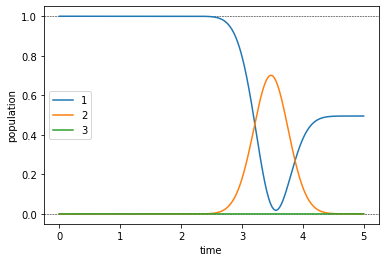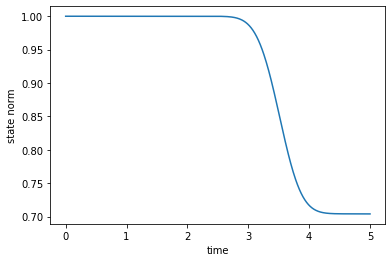The population dynamics and the norm-plot show the effect the non-Hermitian term in the Hamiltonian, resulting in a 30% loss.

## Optimize¶

For each control, we define the update shape and the $\lambda_a$ parameter that determines the magnitude of the update:

In :
def S(t):
"""Scales the Krotov methods update of the pulse value at the time t"""
return krotov.shapes.flattop(
t, t_start=0.0, t_stop=5.0, t_rise=0.3, func='sinsq'
)

In :
pulse_options = {
H: dict(lambda_a=2.0, update_shape=S),
H: dict(lambda_a=2.0, update_shape=S),
H: dict(lambda_a=2.0, update_shape=S),
H: dict(lambda_a=2.0, update_shape=S)
}


We now run the optimization for 40 iterations, printing out the fidelity

$$F_{\text{re}} = \Re{\Braket{\Psi(T)}{\Psi^{\tgt}}}$$

after each iteration.

In :
def print_fidelity(**args):
F_re = np.average(np.array(args['tau_vals']).real)
print("   F = %f" % F_re)
return F_re

In :
oct_result = krotov.optimize_pulses(
objectives, pulse_options, tlist,
propagator=krotov.propagators.expm,
chi_constructor=krotov.functionals.chis_re,
info_hook=print_fidelity,
iter_stop=40
)

   F = -0.007812
F = 0.055166
F = 0.117604
F = 0.178902
F = 0.238507
F = 0.295926
F = 0.350749
F = 0.402648
F = 0.451388
F = 0.496822
F = 0.538882
F = 0.577573
F = 0.612961
F = 0.645161
F = 0.674324
F = 0.700629
F = 0.724268
F = 0.745445
F = 0.764364
F = 0.781226
F = 0.796224
F = 0.809541
F = 0.821349
F = 0.831809
F = 0.841064
F = 0.849250
F = 0.856486
F = 0.862881
F = 0.868532
F = 0.873527
F = 0.877942
F = 0.881847
F = 0.885302
F = 0.888362
F = 0.891074
F = 0.893481
F = 0.895618
F = 0.897519
F = 0.899211
F = 0.900721
F = 0.902071


We look at the optimized controls and the population dynamics they induce:

In :
def plot_pulse_amplitude_and_phase(pulse_real, pulse_imaginary,tlist):
ax1 = plt.subplot(211)
ax2 = plt.subplot(212)
amplitudes = [np.sqrt(x*x + y*y) for x,y in zip(pulse_real,pulse_imaginary)]
phases = [np.arctan2(y,x)/np.pi for x,y in zip(pulse_real,pulse_imaginary)]
ax1.plot(tlist,amplitudes)
ax1.set_xlabel('time')
ax1.set_ylabel('pulse amplitude')
ax2.plot(tlist,phases)
ax2.set_xlabel('time')
ax2.set_ylabel('pulse phase (π)')
plt.show()

print("pump pulse amplitude and phase:")
plot_pulse_amplitude_and_phase(
oct_result.optimized_controls, oct_result.optimized_controls, tlist)
print("Stokes pulse amplitude and phase:")
plot_pulse_amplitude_and_phase(
oct_result.optimized_controls, oct_result.optimized_controls, tlist)

pump pulse amplitude and phase: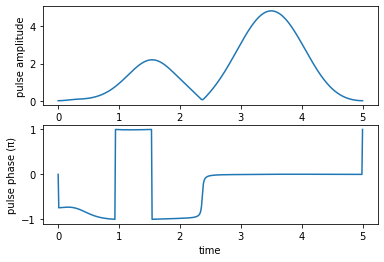Stokes pulse amplitude and phase: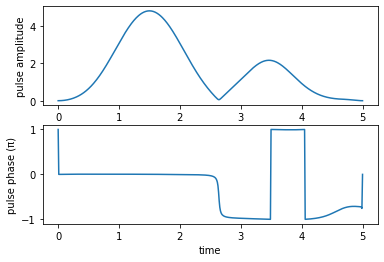We check the evolution of the population due to our optimized pulses.

In :
opt_dynamics = oct_result.optimized_objectives.propagate(
tlist, propagator=krotov.propagators.expm, e_ops=[proj1, proj2, proj3])
opt_states = oct_result.optimized_objectives.propagate(
tlist, propagator=krotov.propagators.expm)

In :
plot_population(opt_dynamics)
plot_norm(opt_states)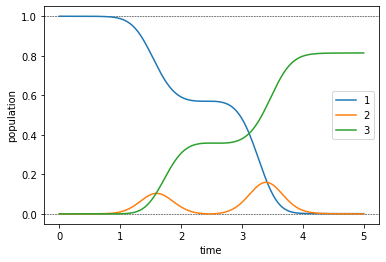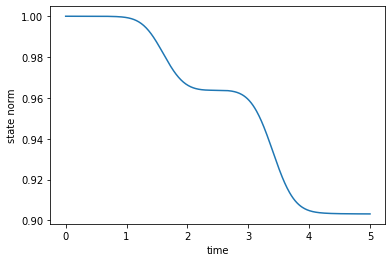These dynamics show that the non-Hermitian Hamiltonian has the desired effect: The population is steered out of the decaying $\ket{2}$ state, with the resulting loss in norm down to 10% from the 30% loss of the guess pulses. Indeed, these 10% are exactly the value of the error $1 - F_{\text{re}}$, indicating that avoiding population in the $\ket{2}$ part is the difficult part of the optimization. Convergence towards this goal is slow, so we continue the optimization up to iteration 2000.

In :
dumpfile = "./non_herm_oct_result.dump"
if os.path.isfile(dumpfile):
else:
oct_result = krotov.optimize_pulses(
objectives, pulse_options, tlist,
propagator=krotov.propagators.expm,
chi_constructor=krotov.functionals.chis_re,
info_hook=krotov.info_hooks.chain(print_fidelity),
iter_stop=2000,
continue_from=oct_result
)
oct_result.dump(dumpfile)

In :
print("Final fidelity: %.3f" % oct_result.info_vals[-1])

Final fidelity: 0.966

In :
def plot_convergence(result):
fig, ax = plt.subplots()
ax.semilogy(result.iters, 1-np.array(result.info_vals))
ax.set_xlabel('OCT iteration')
ax.set_ylabel('error')
plt.show(fig)


To get a feel for the convergence, we can plot the optimization error over the iteration number:

In :
plot_convergence(oct_result)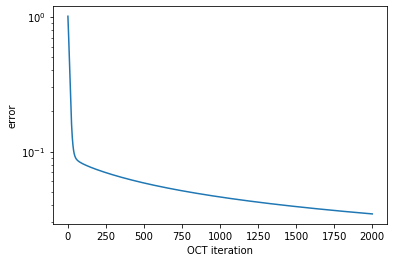We have used here that the return value of the routine print_fidelity that was passed to the optimize_pulses routine as an info_hook is automatically accumulated in result.info_vals.

We also look at optimized controls and the dynamics they induce:

In :
print("pump pulse amplitude and phase:")
plot_pulse_amplitude_and_phase(
oct_result.optimized_controls, oct_result.optimized_controls, tlist)
print("Stokes pulse amplitude and phase:")
plot_pulse_amplitude_and_phase(
oct_result.optimized_controls, oct_result.optimized_controls, tlist)

pump pulse amplitude and phase: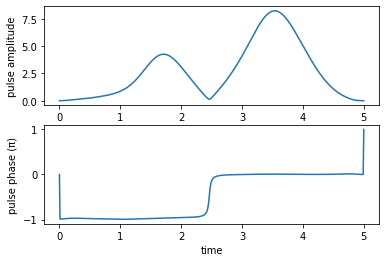Stokes pulse amplitude and phase: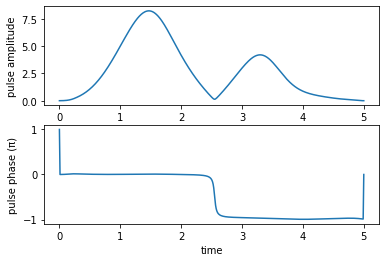In :
opt_dynamics = oct_result.optimized_objectives.propagate(
tlist, propagator=krotov.propagators.expm, e_ops=[proj1, proj2, proj3])
opt_states = oct_result.optimized_objectives.propagate(
tlist, propagator=krotov.propagators.expm)

In :
plot_population(opt_dynamics)
plot_norm(opt_states)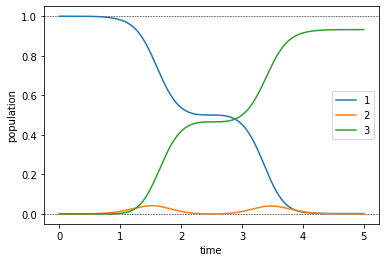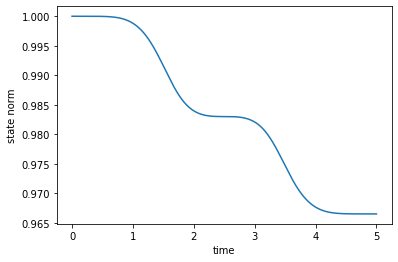In accordance with the lower optimization error, the population dynamics now show a reasonably efficient transfer, and a significantly reduced population in state $\ket{2}$.

Finally, we can convert the complex-valued $\Omega_P$ and $\Omega_S$ functions to the physical electric fields $\epsilon_{P}$ and $\epsilon_{S}$:

In :
def plot_physical_field(pulse_re, pulse_im, tlist, case=None):

if case == 'pump':
w = 9.5
elif case == 'stokes':
w = 4.5
else:
print('Error: selected case is not a valid option')
return

ax = plt.subplot(111)
ax.plot(tlist,pulse_re*np.cos(w*tlist)-pulse_im*np.sin(w*tlist), 'r')
ax.set_xlabel('time', fontsize = 16)
if case == 'pump':
ax.set_ylabel(r'$\mu_{12}\,\epsilon_{P}$')
elif case == 'stokes':
ax.set_ylabel(r'$\mu_{23}\,\epsilon_{S}$')
plt.show()

print('Physical electric pump pulse in the lab frame:')
plot_physical_field(
oct_result.optimized_controls, oct_result.optimized_controls, tlist, case = 'pump')

print('Physical electric Stokes pulse in the lab frame:')
plot_physical_field(
oct_result.optimized_controls, oct_result.optimized_controls, tlist, case = 'stokes')

Physical electric pump pulse in the lab frame: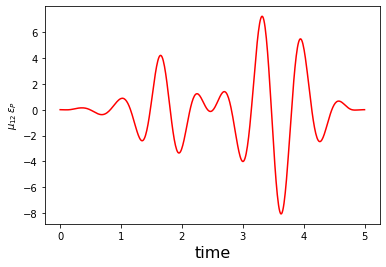Physical electric Stokes pulse in the lab frame: